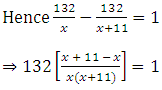##### Get a free home demo of LearnNext

Available for CBSE, ICSE and State Board syllabus.
Call our LearnNext Expert on 1800 419 1234 (tollfree)
OR submit details below for a call back

clear

Gajaanan Muley

Nov 3, 2013

# An express train takes 1 hour less than a passenger train

An express train takes 1 hour less than a passenger train to travel 132 km/hr between Mysore and Bangalore.If the average speed of the express train is 11 km/hr more than that of passenger train,form an equation to find the average speed of the express train.

Kishore Kumar

Let the speed of passenger train be x km/hr Hence speed of express train = (x +11) km/hr Distance travelled by both the trains = 132 km Time taken = (Distance)/(Speed) Time taken by the passenger train = 132/x hrs Time taken by the express train = 132/(x + 11) hrs⇒ 132 × 11 = x(x + 11) ⇒ x2 + 11x – 1452 = 0 ⇒ x2 + 44x – 33x – 1452 = 0 ⇒ x(x + 44) – 33(x + 44) = 0 ⇒ (x + 44)(x – 33) = 0 ⇒ (x + 44) = 0 or (x – 33) = 0 ∴ x = –44 or x = 33 Since speed cannot be negative, x = 33 Thus the sped of passenger train is 33 km/hr and speed of express train is 44 km/hr.

Agam Gupta

Let the speed of passenger train be x km/hr Hence speed of express train = (x +11) km/hr Distance travelled by both the trains = 132 km Time taken = (Distance)/(Speed) Time taken by the passenger train = 132/x hrs Time taken by the express train = 132/(x + 11) hrs⇒�132 × 11 = x(x + 11) ⇒�x2�+ 11x – 1452 = 0 ⇒�x2�+ 44x – 33x – 1452 = 0 ⇒�x(x + 44) – 33(x + 44) = 0 ⇒�(x + 44)(x – 33) = 0 ⇒�(x + 44) = 0 or (x – 33) = 0 ∴ x = –44 or x = 33 Since speed cannot be negative, x = 33 Thus the sped of passenger train is 33 km/hr and speed of express train is 44 km/hr.
###### Like NextGurukul? Also explore our advanced self-learning solution LearnNext
Offered for classes 6-12, LearnNext is a popular self-learning solution for students who strive for excellence
Explore
Animated Video
lessons
All India
Test Series
Interactive Video
Experiments
Best-in class
books